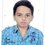# INMO 2017 Board

$$(1)$$ Let $${ x }_{ 1 },...,{ x }_{ 2017 }$$ be positive reals such that

$\huge\ \frac { 1 }{ { x }_{ 1 }+2017 } +\frac { 1 }{ { x }_{ 2 }+2017 } +...+\frac { 1 }{ { x }_{ 2017 }+2017 } =\frac { 1 }{ 2017 }$

Prove that

$\huge\ \frac { \sqrt [ 2017 ]{ { x }_{ 1 }{ x }_{ 2 }...{ x }_{ 2017 } } }{ 2016 } \ge 2017$

Two circles enclose non-intersecting areas. Common tangent lines to the two circles, one external and one internal, are drawn. Consider two straight lines each of which passes through the tangent points on one of the circles. Prove that the intersection point of the lines lies on the straight line that connects the centers of the circles.

Hello everybody. Please post solutions of these problems and post problems on your own also.

These are sample problems.Note by Priyanshu Mishra
4 years, 9 months ago

This discussion board is a place to discuss our Daily Challenges and the math and science related to those challenges. Explanations are more than just a solution — they should explain the steps and thinking strategies that you used to obtain the solution. Comments should further the discussion of math and science.

When posting on Brilliant:

• Use the emojis to react to an explanation, whether you're congratulating a job well done , or just really confused .
• Ask specific questions about the challenge or the steps in somebody's explanation. Well-posed questions can add a lot to the discussion, but posting "I don't understand!" doesn't help anyone.
• Try to contribute something new to the discussion, whether it is an extension, generalization or other idea related to the challenge.

MarkdownAppears as
*italics* or _italics_ italics
**bold** or __bold__ bold
- bulleted- list
• bulleted
• list
1. numbered2. list
1. numbered
2. list
Note: you must add a full line of space before and after lists for them to show up correctly
paragraph 1paragraph 2

paragraph 1

paragraph 2

[example link](https://brilliant.org)example link
> This is a quote
This is a quote
    # I indented these lines
# 4 spaces, and now they show
# up as a code block.

print "hello world"
# I indented these lines
# 4 spaces, and now they show
# up as a code block.

print "hello world"
MathAppears as
Remember to wrap math in $$ ... $$ or $ ... $ to ensure proper formatting.
2 \times 3 $2 \times 3$
2^{34} $2^{34}$
a_{i-1} $a_{i-1}$
\frac{2}{3} $\frac{2}{3}$
\sqrt{2} $\sqrt{2}$
\sum_{i=1}^3 $\sum_{i=1}^3$
\sin \theta $\sin \theta$
\boxed{123} $\boxed{123}$

Sort by:

@Harsh Shrivastava, @Sharky Kesa, @Svatejas Shivakumar, @rajdeep das, @Racchit Jain,@Vaibhav Prasad @Kalash Verma @Nihar Mahajan @Adarsh Kumar @Akshat Sharda @AkshayYadav @Swapnil Das @Rajdeep Dhingra @Anik Mandal @Lakshya Sinha @Abhay Kumar @Dev Sharma and everyone.

Come and enjoy solving problems here.

- 4 years, 9 months ago

For problem 1 apply titus lemma in the constraint given and then apply AM GM and it's done.

- 4 years, 9 months ago

Is RMO DELHI results out?

At which website?

- 4 years, 9 months ago

They send you your marks by mail. Only marks though, the cutoff hasn't been decided yet.

- 4 years, 9 months ago

At which website is the name of list of selected students declared?

- 4 years, 9 months ago

It's not declared yet but I think it will be on hbcse

- 4 years, 8 months ago

Any more problems?

- 4 years, 9 months ago

i HAve added newproblems. TRy them.

- 4 years, 8 months ago

Try the new problems

- 4 years, 8 months ago

Where are the new problems.

- 4 years, 6 months ago

- 4 years, 6 months ago

Problem 1 was just an AM-GM.

Let $y_i = \dfrac {1}{x_i + 2017}$, so $x_i = \dfrac {1 - 2017y_i}{y_i}$

We have

$\displaystyle \sum_{j=1}^{2017} y_j = \dfrac{1}{2017}$

Thus,

\begin{aligned} \dfrac{1}{2017} - y_i &= \displaystyle \sum_{\stackrel{j=1}{j \neq i}}^{2017} y_j\\ 1 - 2017 y_i &= 2017 \displaystyle \sum_{\stackrel{j=1}{j \neq i}}^{2017} y_j\\ \end{aligned}

However, we also have

$\displaystyle \sum_{\stackrel{j=1}{j \neq i}}^{2017} y_j \geq 2016 \left ( \displaystyle \prod_{\stackrel{j=1}{j \neq i}}^{2017} y_j \right ) ^{\dfrac {1}{2016}}$

Therefore,

\begin{aligned} \displaystyle \prod_{i=1}^{2017} x_i &= \displaystyle \prod_{i=1}^{2017} \dfrac {1 - 2017y_i}{y_i}\\ & = \dfrac 2017^{2017} \prod_{i=1}^{2017} \left (\displaystyle \sum_{\stackrel{j=1}{j \neq i}}^{2017} y_j \right )}\prod_{i=1}^{2017} y_i}\\ &\geq \dfrac {(2016 \times 2017)^{2017} \displaystyle \prod_{i=1}^{2017} \left ( \displaystyle \prod_{\stackrel{j=1}{j \neq i}}^{2017} y_j \right )^{\dfrac{1}{2016}}}\prod_{i=1}^{2017} y_i}\\ &= 2016^{2017} \times 2017^{2017}\\ \end{aligned

This just rearranges to give us the desired expression.

- 4 years, 5 months ago